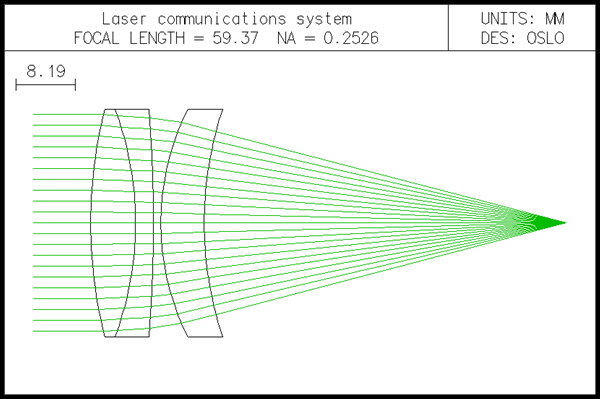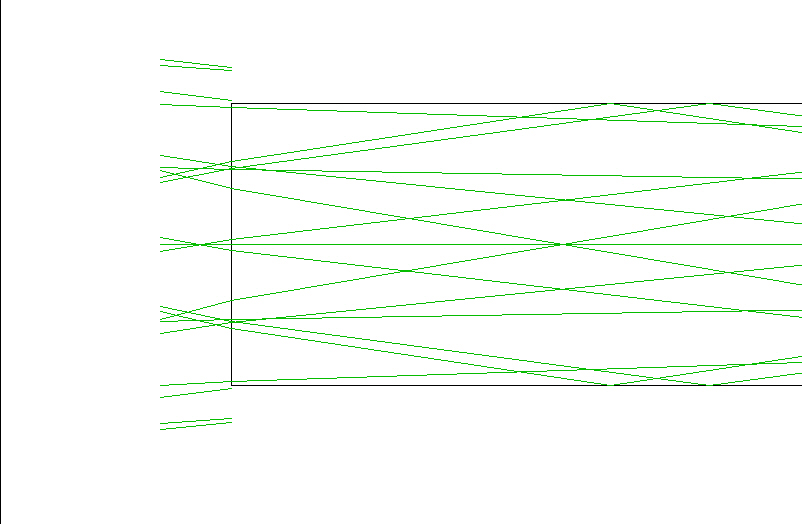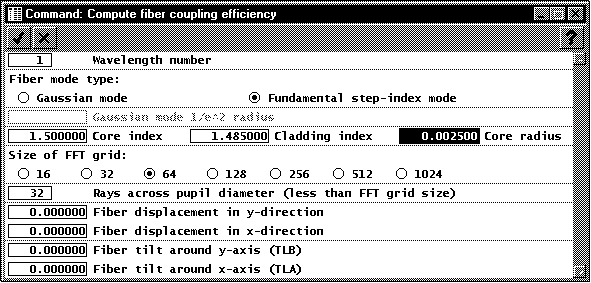# LASRCOMM.LEN - AN APLANATIC LASER FOCUSING SYSTEM

Lasrcomm is the final design of the laser communication system described next. It is combined with a tiny single-mode fiber to show the fiber efficiency calculations that are built into OSLO. This system designed elsewhere using Melles Griot catalog lenses. Here, a medium "light pipe" has been added in the image plane to simulate a fiber. The light pipe has a diameter of 0.005mm, and a length of 0.05mm, so it is similar in geometrical size to the one postulated for the example. To see the fiber, you must make a special drawing in which you limit the surfaces drawn to 6 and 7, then zoom in as much as possible. The result is the second drawing below.You can readily see that the extreme rays miss the edge of the fiber. Of course no particular quantitative information can be obtained from this, since the system is close to the diffraction limit. If you want to obtain detailed information on the coupling into the fiber, you should use the Options >> Fiber coupling command. If you want to use this command, you should first remove the light pipe (surfaces 6 and 7) from the system, since the command assumes that the fiber is located in the image plane. The Source >> Fiber coupling command produces the following dialog box:According to the original problem, the diameter of the fiber core is 5 microns. If we assume a refractive index of 1.5, and a cladding index difference of 1%, the actual coupling efficiency is about -12 dB, as shown by the output below. Evidently the mode coupling into the fiber is not so well optimized as it should be.

*FIBER COUPLING EFFICIENCY – WAVELENGTH 1 FUNDAMENTAL MODE OF STEP-INDEX FIBER

 CORE INDEX = 1.500000 CLADDING INDEX = 1.485000 CORE RADIUS = 0.002500 FIBER DISPLACEMENT Y -- X -- FIBER TILT TLB -- TLA -- POWER COUPLING = 0.0569 (-12.45dB) AMPLITUDE COUPLING REAL = 0.09865 IMAGINARY = 10.2172
The fiber coupling command also gives you the capability to experiment with alignment tolerances. Note however, that reflection losses are not accounted for.